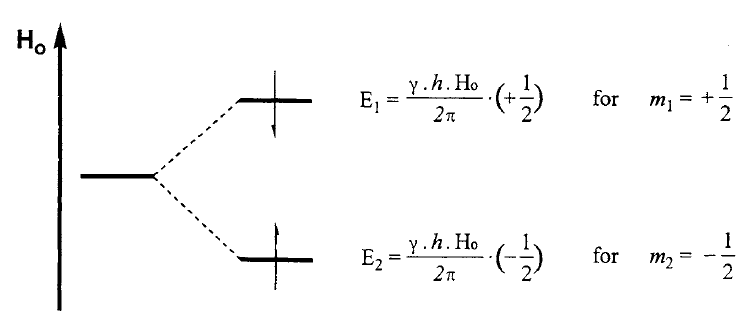# Resonance in NMR

Resonance in Nuclear Magnetic Resonance (NMR) is a fundamental principle that underlies the functioning of this analytical technique. NMR spectroscopy is a powerful tool used to study the structure, dynamics, and composition of molecules at the atomic level. It is widely utilized in various scientific disciplines, including chemistry, biochemistry, physics, and materials science.

NMR relies on the principle of resonance, which is the phenomenon where a system oscillates with maximum amplitude at a particular frequency. In the case of NMR, the system of interest is the atomic nucleus, which possesses a property called spin. When placed in a strong external magnetic field, these spinning nuclei generate a tiny magnetic field of their own. This magnetic field interacts with the external magnetic field and gives rise to resonance.

The key to achieving resonance in NMR is to apply a radio frequency (RF) pulse that matches the energy difference between the nuclear spin states. Nuclei can exist in different spin states, typically represented as spin "up" and spin "down." The energy difference between these states is determined by the external magnetic field strength, and it is proportional to the resonant frequency, which is usually in the radio frequency range.

For example, proton and carbon nucleus have spin quantum number I=1/2 and so there are two energy states. The lower energy states consists of nuclei which have magnetic quantum m = -1/2 and are oriented parallel to the magnetic field and have spin up states. Similarly the higher energy sates consists of nuclei which have magnetic quantum number m=1/2, are oriented anti-parallel to the magnetic field and have spin down states. This is illustrated in the diagram below.The two energy levels are below,

$$E_1 = \frac{\gamma h H_0}{2 \pi} (+\frac{1}{2})$$

and, $$E_2 = \frac{\gamma h H_0}{2 \pi} (-\frac{1}{2})$$

See previous post how to derive Energy Levels in NMR

When the RF pulse is applied at the resonant frequency, it imparts energy to the system, causing the nuclei to transition between the spin states. This energy transfer is only efficient at the resonant frequency because it matches the energy difference between the states. In other words, the RF pulse selectively excites the nuclei that resonate with its frequency.

The energy difference is,

$$\Delta E = E_1-E_2 = \frac{\gamma h H_0}{2 \pi} (+\frac{1}{2}) - \frac{\gamma h H_0}{2 \pi} (-\frac{1}{2}) = \frac{\gamma h H_0}{2 \pi}$$

So the RF energy required is,

$$E = h f$$

where $$f$$ is the RF signal frequency in MHz.

Hence $$E = h f = \frac{\gamma h H_0}{2 \pi}$$

or,  $$f = \frac{\gamma H_0}{2 \pi}$$

which represents the equation for resonance frequency in NMR.

Such RF signal can be obtained from various RF oscillator circuits.

After the excitation, the nuclei return to their original spin states, releasing the absorbed energy. This energy is detected by a receiver coil in the NMR spectrometer, and it provides valuable information about the sample being studied. The detected signal is then converted into a spectrum, which represents the frequencies and intensities of the resonating nuclei.

The chemical environment surrounding the nuclei affects the resonant frequency and, consequently, the NMR spectrum. Different chemical compounds exhibit distinct resonances due to variations in electron distribution, molecular structure, and interatomic interactions. By analyzing these resonances, scientists can deduce valuable information about molecular structures, such as the connectivity of atoms, stereochemistry, and chemical environments.

Resonance in NMR is also influenced by various factors, including the strength and homogeneity of the magnetic field, the nature of the sample, temperature, and relaxation processes. Careful calibration and optimization of these factors are essential for obtaining high-quality NMR spectra.

In summary, resonance is a central concept in NMR spectroscopy, allowing scientists to selectively excite and detect nuclear spins in a sample. By exploiting the resonant behavior of nuclei, NMR provides valuable insights into molecular structure, dynamics, and interactions, making it an indispensable tool in modern scientific research and various practical applications.